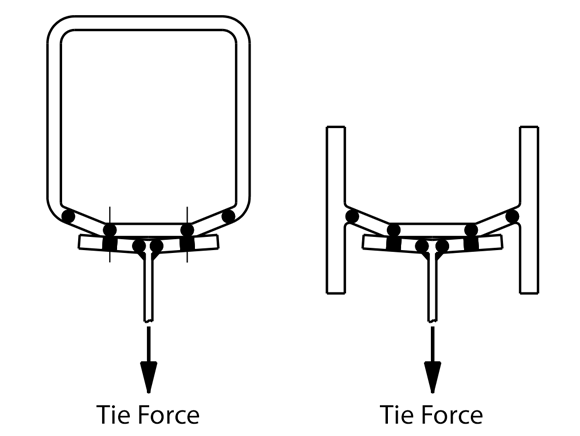# AD 396: Tying resistance of flexible end plates in one-sided connections

When calculating the resistance of a flexible end plate under a tying force, the design checks in the Green Books (Check 11 in SCI P212, 2009 and Check 11 in SCI P358, 2014) assume in every case that the end plate will deform in double curvature bending, as shown in Figure 1.Figure 1: Assumed behaviour of an end plate under a tying force

The assumption that the end plate is in double curvature bending may be recognised by the form of the resistance equations; for P358 it can be compared to the expressions in Table 6.2 of BS EN 1993-1-8:2005. The equations are from the part of the table covering situations when prying forces may develop – i.e. the plate resistance is determined assuming double curvature bending.

When end plates are connected to a hollow section, or to one side (only) of a web, the assumption that prying can develop appears optimistic. As shown in Figure 2, the end plate may separate from the supporting member, and no prying occurs. In these circumstances, the expressions in Table 6.2 of BS EN 1993-1-8:2005 for “No prying” would appear to be more appropriate, which would mean a considerable reduction in resistance.Figure 2: Behaviour in one-sided connections to webs and hollow sections

SCI have completed a series of Finite Element analyses investigating the behaviour of one-sided connections to webs and connections to hollow sections. The study found that when the supporting element (web or hollow section wall) is relatively thin, no prying occurs. Despite there being no prying force, the resistance calculated assuming prying occurs is still conservative. The study showed that there is considerable yielding of the plates around the bolt, due to the clamping action between bolt head and nut. This yielding is ignored in the simple expression presented in the Eurocode for the “no prying” situations.

The study concluded that it remains appropriate to use the rules in the Green Books (which assume prying and double curvature bending) in all circumstances when calculating the tying resistance of a flexible end plate.

Contact: Abdul Malik
Tel: 01344636525